Quandaries and Queries I am a non-traditional college student who hasn't had geometry since elementary school. I would like to know how to draw different shapes: Regular Octagon with sides of length, 1 unit Equilateral Triangles, with sides of length 1 unit Regular Hexagons, with sides of length 1 unit Isoseles Triangles, with hypotenuse of length 1 unit (I figured out the square) Thank you Kim Hi Kim, By draw I assume you mean that you have to construct these shapes with a straight edge and a compass. To construct a regular octagon you need to construct a 45 degree angle.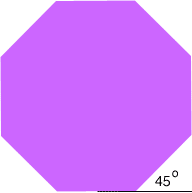If you draw a diagonal in the square you have already constructed then you have a 45 degree angle.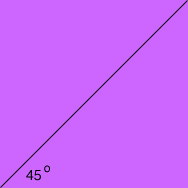For both an equilateral triangle and a regular hexagon you need to construct a 60 degree angle. An equilateral triangle, and hence a 60 degree angle can be construct as follows. Draw a line segment and call its length the unit length. I am going to label the ends of the line A and B so that I can refer to them.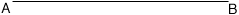Place the point of the compass at A and the point of the pencil on the other arm of the compass at B. Keeping the point of the compass at A draw an arc above the center of the line segment.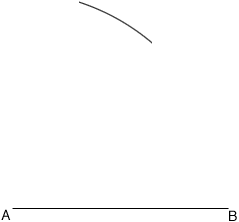Lift the compass and place the point at B being careful not to change the distance between its ends. Use the pencil end of the compass to draw an arc, intersecting the arc drawn in step 3. Call this intersection point P.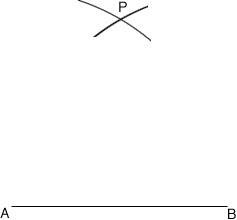Join A to P and B to P. The lengths |AB|, |BP| and |PA| are equal and hence the triangle PAB is an equilateral triangle and the angles PAB, ABP an BPA are each 60 degree angles. In your last problem I assume you want an isosceles right angles triangles, with hypotenuse of length 1 unit. One of the figures you have already drawn can be cut up into pieces that are isosceles right angles triangles with hypotenuse of length 1 unit. Penny